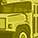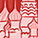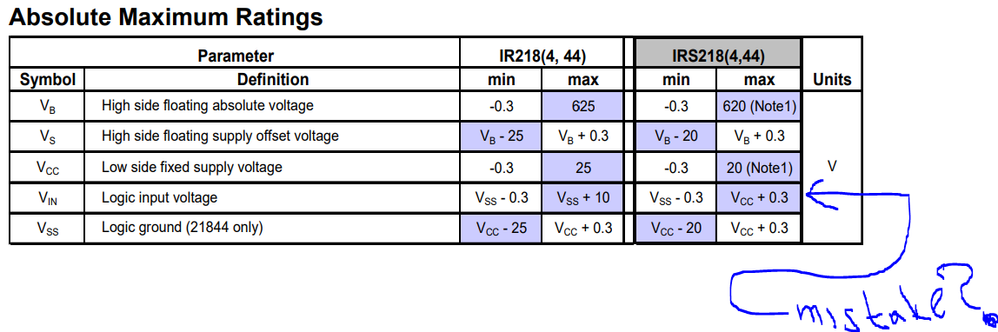# Gate Driver ICs Forum DiscussionsLevel 1
Level 1

# IR2214 component calculations assistance

Hello all,

I am currently working with a PCB we have designed as a 3 phase inverter to do FOC control of a BLDC motor, aiming for 15kW (10KHz switching).

I am working through the calculations for the gate resistances but it isnt particularly clear where I obtain the total switching time for Rgon?? I have assumed that this is the sum of tr, tf, td(on) and td(off) from the IGBT datasheet?? (We are using 6x STGW40M120DF3 for the whole thing).

The design incorporates the optional gate resistor for the soft shutdown aswell as the series resistor at Vs and COM, I cant seem to find how these are calculated either? 🙂

The isolated power supply which is powering VDD on the three drivers is 2W, and Im also wondering if this is enough to run the three half-bridges sufficiently.

Any tips/pointers for this IC would be much appreciated.

Thanks all!

EDIT: I have grabbed the values from my spreadsheet to see if they check out:

For the above IGBTs (using 15V for VDD) I have calculated the following for the Rgon and Rgoff gate resistors, Rgon=4.863R (probably use 5R), Rgoff(max)=13.056R (Probably use 12R). I used a figure of 325ns for Tsw based on the above assumption.
1 SolutionModeratorModerator

# Re: IR2214 component calculations assistance

You can use the below approach to implement an active Miller clamp using a PNP transistor.3 RepliesModeratorModerator

# Re: IR2214 component calculations assistance

Gate resistor calculations are explained in pages 17 to 19 of IR2214 datasheet.

The power dissipation calculations are available at https://www.infineon.com/dgdl/Infineon-EiceDRIVER-Gate_resistor_for_power_devices-ApplicationNotes-v...

For Rgon of 5R and Rgoff of 12R and internal Ron of 15R from gate driver, the drive current is Vdd / Rtotal = 15 / 20 = 0.75 A for turn on and 0.55 A for turn off. This is not exceeding the IC capability.

Pdon = 0.5 * Qg * Vcc*fsw *Ron/(Ron+Rgon)
Pdoff = 0.5 * Qg * Vcc*fsw*Roff/(Roff+Rgoff)
Pdstatic = Vcc*Istatic
Pdtotal < Pd of 1.5W as per device datasheet. As per above you are well below this.

Soft shutdown on resistance is specified on page 4 as 90R based on the desat timing requirement.

2W is sufficient to run the three half bridges. Total energy to drive per IGBTs = 0.5 Cgs * VDD*VDD ~ 3 uJ, power required = 3 uJ * 10 kHz = 30 mW x 6 = 180 mW ~ 12 mA.Level 1
Level 1

# Re: IR2214 component calculations assistance

Hi again all,

We have a solution working now on our system however we appear to be damaging our low side IGBTs when we increase the load on the motor (not by much).

The low side IGBT is ALWAYS the one to become faulty, it is random which phase it happens to.

We have managed to scope the gate voltage and can see a pulse on the line when we see the high side IGBT turn on, and also when the other phases are energising.........this appears at various positions when the low side gate is in its on state (i.e. at 15V).

Our thought was to first try adding some extra capacitance across the gate of each IGBT (10nF) which has reduced the amplitude of the pulses quite a bit, and also reducing the breakdown voltage of a TVS we have across the gate as well.

We have looked at the possibility of an active miller clamp (unfortunately the IR2214SS doesn't have this feature) and were wondering how we would go about implementing this in circuit alongside the IR2214SS??

Any advice would be much appreciated.

Best Regards,ModeratorModerator

# Re: IR2214 component calculations assistance